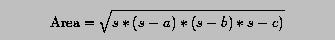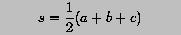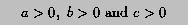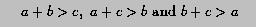# Heron's Formula for Computing Triangle Area - Revisited### Problem Statement

Given a triangle with side lengths a, b and c, its area can be computed using the Heron's formula:where s is the half of the perimeter length:In order for a, b and c to form a triangle, two conditions must be satisfied. First, all side lengths must be positive:Second, the sum of any two side lengths must be greater than the third side length:Write a program to read in three real values and use a function for testing the conditions and another function for computing the area. Should the conditions fail, your program must keep asking the user to re-enter the input until the input form a triangle. Then, the other function is used to compute the area.### Solution

```! --------------------------------------------------------------------
!    This program uses Heron's formula to compute the area of a
! triangle.  It "contains" the following functions;
!    (1)  LOGICAL function TriangleTest() -
!         this function has three real formal arguments and tests
!         to see if they can form a triangle.  If they do form a
!         triangle, this function returns .TRUE.; otherwise, it
!         returns .FALSE.
!    (2)  REAL function TriangleArea() -
!         this functions has three real formal arguments considered
!         as three sides of a triangle and returns the area of this
!         triangle.
! --------------------------------------------------------------------

PROGRAM  HeronFormula
IMPLICIT  NONE

REAL :: a, b, c, TriangleArea

DO
WRITE(*,*)  'Three sides of a triangle please --> '
READ(*,*)   a, b, c
WRITE(*,*)  'Input sides are ', a, b, c
IF (TriangleTest(a, b, c))  EXIT  ! exit if not a triangle
WRITE(*,*)  'Your input CANNOT form a triangle.  Try again'
END DO

TriangleArea = Area(a, b, c)
WRITE(*,*)  'Triangle area is ', TriangleArea

CONTAINS

! --------------------------------------------------------------------
! LOGICAL FUNCTION  TriangleTest() :
!    This function receives three REAL numbers and tests if they form
! a triangle by testing:
!    (1)  all arguments must be positive, and
!    (2)  the sum of any two is greater than the third
! If the arguments form a triangle, this function returns .TRUE.;
! otherwise, it returns .FALSE.
! --------------------------------------------------------------------

LOGICAL FUNCTION  TriangleTest(a, b, c)
IMPLICIT  NONE

REAL, INTENT(IN) :: a, b, c
LOGICAL          :: test1, test2

test1 = (a > 0.0) .AND. (b > 0.0) .AND. (c > 0.0)
test2 = (a + b > c) .AND. (a + c > b) .AND. (b + c > a)
TriangleTest = test1 .AND. test2  ! both must be .TRUE.
END FUNCTION  TriangleTest

! --------------------------------------------------------------------
! REAL FUNCTION  Area() :
!    This function takes three real number that form a triangle, and
! computes and returns the area of this triangle using Heron's formula.
! --------------------------------------------------------------------

REAL FUNCTION  Area(a, b, c)
IMPLICIT  NONE

REAL, INTENT(IN) :: a, b, c
REAL             :: s

s    = (a + b + c) / 2.0
Area = SQRT(s*(s-a)*(s-b)*(s-c))
END FUNCTION  Area

END PROGRAM  HeronFormula
```
Click here to download this program.### Program Input and Output

The following is the output from the above program.
```Three sides of a triangle please -->
-3.0  4.0  5.0
Input sides are -3.,  4.,  5.
Your input CANNOT form a triangle.  Try again
Three sides of a triangle please -->
1.0  3.0  4.0
Input sides are 1.,  3.,  4.
Your input CANNOT form a triangle.  Try again
Three sides of a triangle please -->
6.0  8.0  10.0
Input sides are 6.,  8.,  10.
Triangle area is 24.
```### Discussion

• LOGICAL function TriangleTest() receives three REAL values. The result of the first test condition is saved to a local LOGICAL variable test1, while the result of the second condition is saved to another LOGICAL variable test2. Since both conditions must be true to have a triangle, test1 and test2 are .AND.ed and the result goes into the function name so that it could be returned.
• REAL function Area is simple and does not require further discussion. However, please note that Area() has three formal arguments whose names are identical to the three global variables declared in the main program. By Scope Rule 3, they are different entities and do not cause any conflicts.
• The main program has a DO-EXIT-END DO loop. In each iteration, it asks for three real values. These values are sent to LOGICAL function TriangleTest() for testing. If the returned value is .TRUE., the input form a triangle and the control of execution exits. Then, the area is computed with REAL function Area(). If the returned value is .FALSE., this function displays a message and goes back asking for a new set of values.